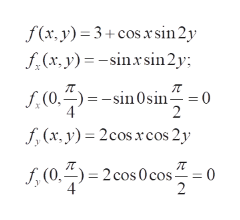# Let f(x,y)= 3+cos(x) sin(2y)a) Show that the function has a stationary point at (0,Pi/4).b) Is (0, Pi/4) a local maximum, minimum or saddle point? Justify your answer mathematically.

Question
54 views

Let f(x,y)= 3+cos(x) sin(2y)

a) Show that the function has a stationary point at (0,Pi/4).

check_circle

Step 1

To analyze the critical point (0,pi/4) of the given function of two variables

Step 2

By definition, a stationary point is where both partial derivatives are 0.  a) is verified ,as shown. (suffixes denote partial derivatives).help_outlineImage Transcriptionclosef(x, y) 3+cos xsin 2y f(x, y)-sinsin 2y f,(0,)sin0sin = 0 2 f,(x, y) 2cosxrcos 2y (0, 2cos 0 cos= 0 2 fullscreen
Step 3

b) to analyze further, we need the criter...

### Want to see the full answer?

See Solution

#### Want to see this answer and more?

Solutions are written by subject experts who are available 24/7. Questions are typically answered within 1 hour.*

See Solution
*Response times may vary by subject and question.
Tagged in

### Derivative